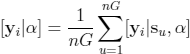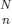Ecological Archives E094-022-A1

J. Andrew Royle, Richard B. Chandler, Kimberly D. Gazenski, Tabitha A. Graves. 2013. Spatial capture–recapture models for jointly estimating population density and landscape connectivity. Ecology 94:287–294. http://dx.doi.org/10.1890/12-0413.1

Appendix A. Details for computing the marginal likelihood and obtaining the maximum likelihood estimators (MLEs).

The key operation for computing the likelihood is solving the 2-dimensional integration problem to remove s. There are some general purpose R packages that implement a number of multi-dimensional integration routines including R2Cuba (Hahn et al., 2010). We won't rely on these extraneous R packages but instead will use perhaps less efficient methods in which we replace the integral with a summation over an equal area mesh of points on the state-space S and explicitly evaluate the integrand at each point. We invoke the rectangular rule for integration here in which the integrand is evaluated on a regular grid of points of equal area and then averaged. Let u = 1, 2, ..., nG index a grid of nG points, su, where the area of grid cell u is constant. In this case, the integrand, i.e., the marginal pmf of yi, is approximated by:(A.1)

To deal with the fact that N is unknown, there are two key issues that need to be addressed. First is that we don't observe the "all-zero" encounter histories (i.e., yij = 0 for all j) corresponding to uncaptured individuals, so we have to make sure we compute the probability for that all zero encounter history which we do operationally by tacking a row of zeros onto the encounter history matrix. We include the number of such all-zero encounter histories as an unknown parameter of the model, which we label n0. In addition, we have to be sure to include a combinatorial term to account for the fact that of the n observed individuals there are () ways to realize a sample of size n. The combinatorial term involves the unknown n0 and thus it must be included in the likelihood.

To compute the integral requires that the bounds of integration are specified, which is equivalent to prescribing the state-space of the underlying point process, i.e., S. Given S, density is computed as D(S) = N/||S||. In our simulation study below we report N as the two are equivalent summaries of the data set once the state-space is fixed.

We wrote an R function to evaluate the likelihood which we optimize using the R function optim (or, alternatively, nlm).

Literature Cited

Hahn, T., A. Bouvier, and K. Kiêu, 2010. R2Cuba: Multidimensional Numerical Integration. R package version 1.0-6.